2020-03-18 10:10:57

## 你知道如何从海量字符串中快速找到指定内容吗？

32 / 0 / 0 / 0

/   今日科技快讯   /

/   作者简介   /

https://www.jianshu.com/u/dbae9ac95c78

/   前言   /

/   朴素模式匹配算法   /

/*****
* Java代码实现朴素模式匹配
*
* @param stringS 主串S
* @param stringT 模式串T
*/
public boolean match(String stringS, String stringT) {
char[] charsT = stringT.toCharArray();
for (int i = 0, sizeI = charsS.length - charsT.length; i <= sizeI; i ++) {
for (int j = 0, sizeJ = charsT.length; j < sizeJ; j ++) {
if (charsS[i + j] != charsT[j]) {
break;
}
if (sizeJ - 1 == j) {
return true;
}
}
}
return false;
}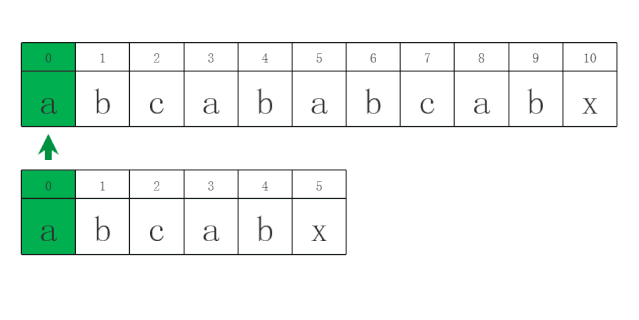/   KMP模式匹配算法   /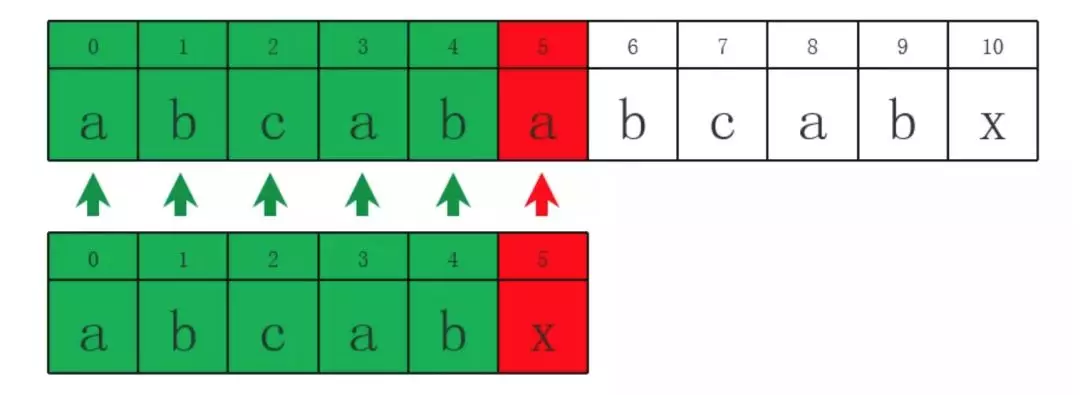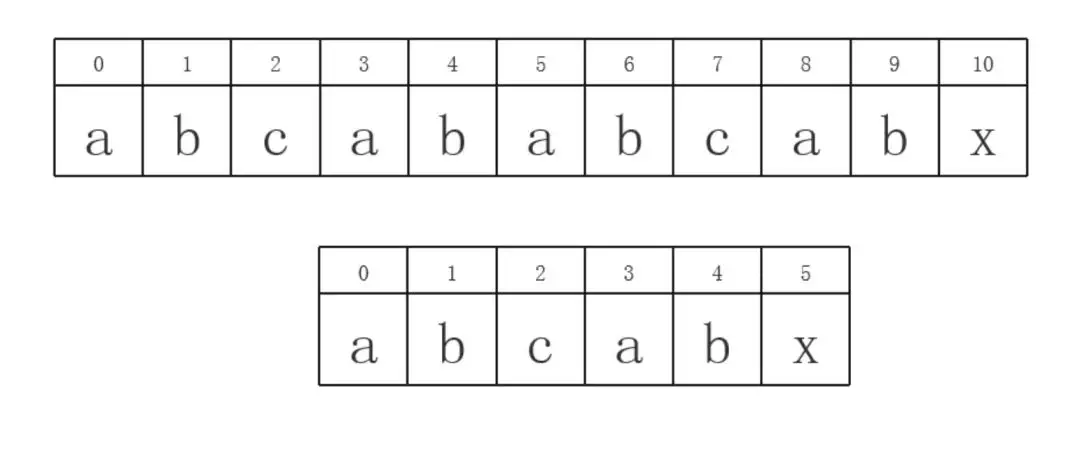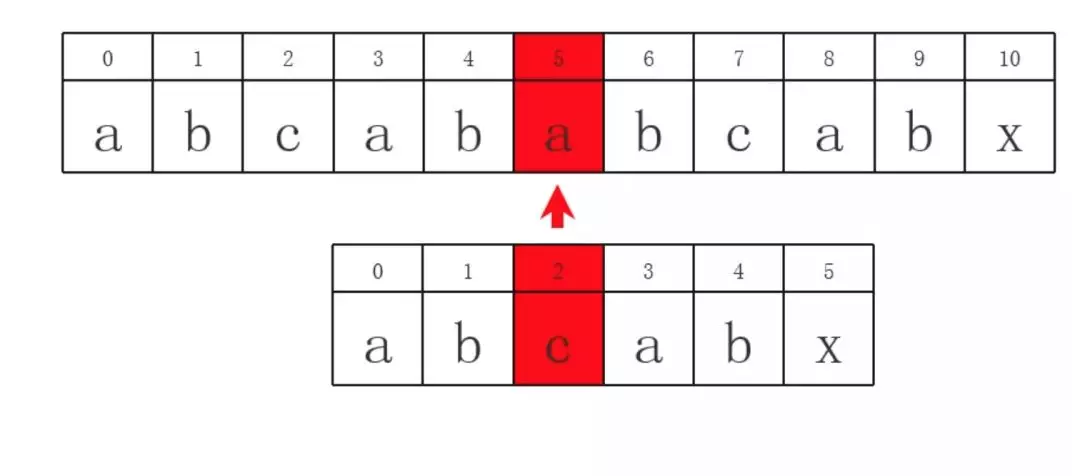/   KMP算法-next[]数组推导   /

KMP算法就是根据模式串的字符前后位置信息生成一个与模式串等长的整形数组next[]，用来存储当模式串上每个位置上的字符如果和主串所对应字符不相同的时候，根据next[]中得到的整型数值作为指引，做一个最优的滑动，什么叫最优滑动呢？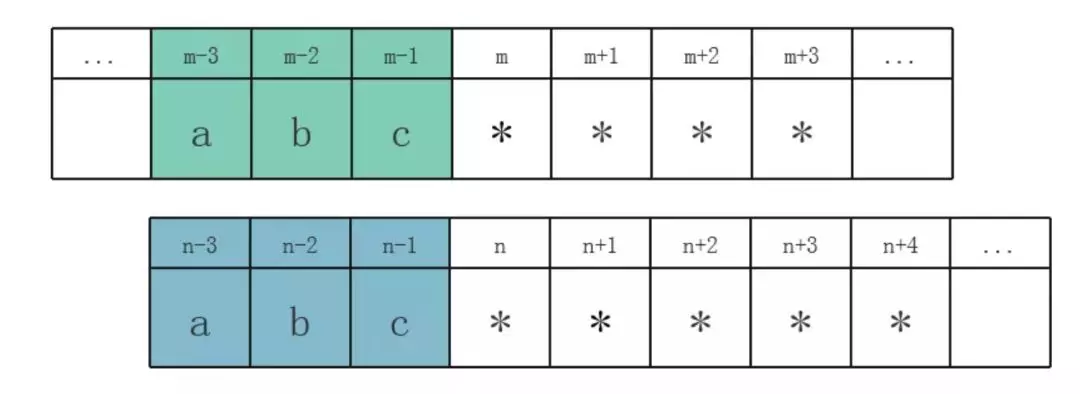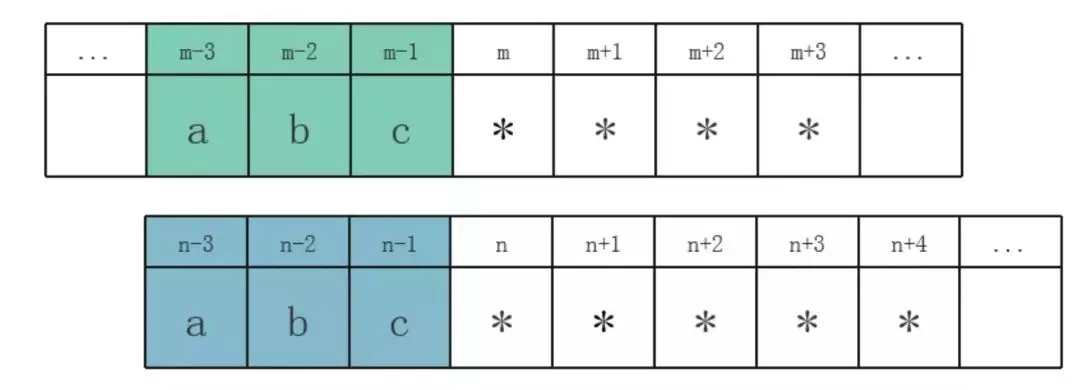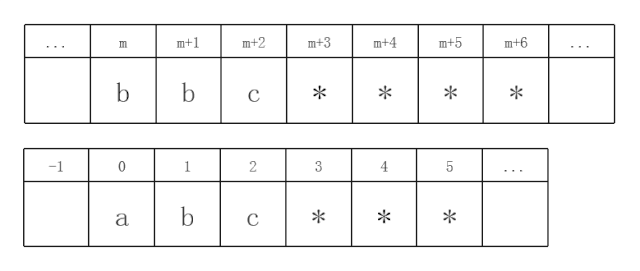/**
* Java代码实现KMP模式匹配算法next[]推导过程
*
* @param stringT 模式串
* */
private int[] getNext(String stringT) {
char[] chars = stringT.toCharArray();
int[] next = new int[chars.length];
next = -1;  // 这是一个必然的结果，不管是对什么模式串，以此为突破点往后推导
for (int i = 1; i < chars.length; i ++) {
int j = next[i - 1];
while (true) {
if (-1 == j || chars[i - 1] == chars[j]) {
next[i] = j + 1;
break;
} else {
j = next[j];
}
}
}
return next;
}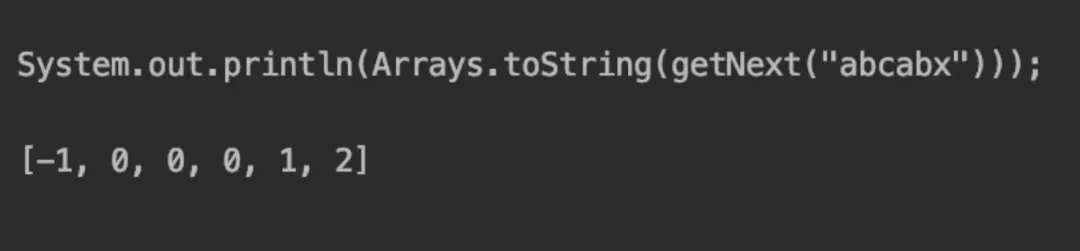/*****
* Java代码实现KMP模式匹配
*
* @param stringS 主串S
* @param stringT 模式串T
*/
public boolean match(String stringS, String stringT) {
char[] charsT = stringT.toCharArray();
int[] next = getNext(stringT);
int j = 0;
for (int i = 0, sizeI = charsS.length; i < sizeI; i ++) {
// 此处0 > j其实就是针对next[j]=-1的情况，跳过主串当前字符的对比
if (0 > j || charsT[j] == charsS[i]) {
j ++;
} else {
j = next[j];
i --; // 实现继续对比主串的同一个位置的字符
}
if (charsT.length == j) {
return true;
}
}
return false;
}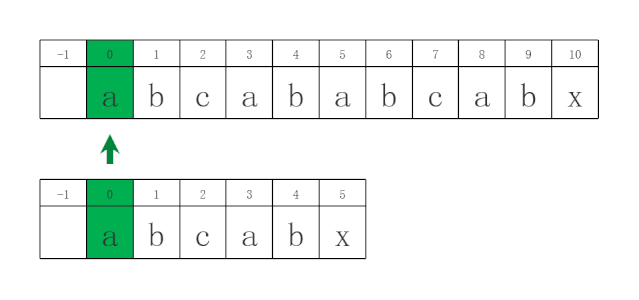/   KMP算法-next[]数组推导优化   /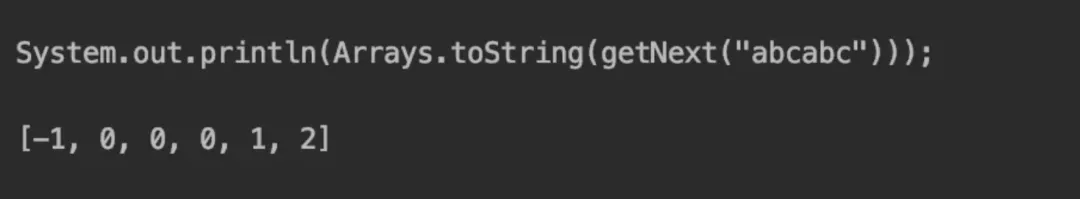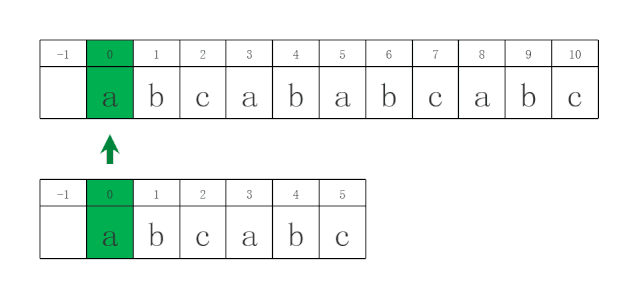/**
* Java代码实现KMP模式匹配算法next[]推导过程
* 针对next[]推荐对比字符相同问题优化
*
* @param stringT 模式串
* */
private int[] getNextPlus(String stringT) {
char[] chars = stringT.toCharArray();
int[] next = new int[chars.length];
next = -1; // 这是一个必然的结果，不管是对什么模式串，以此为突破点往后推导
for (int i = 1; i < chars.length; i ++) {
int j = next[i - 1];
while (true) {
if (-1 == j || chars[i - 1] == chars[j]) {
next[i] = j + 1;
break;
} else {
j = next[j];
}
}
}

// 优化版增添代码
for (int i = 0; i < next.length; i ++) {
if (0 <= next[i] && chars[next[i]] == chars[i]) {
next[i] = next[next[i]];
}
}
return next;
}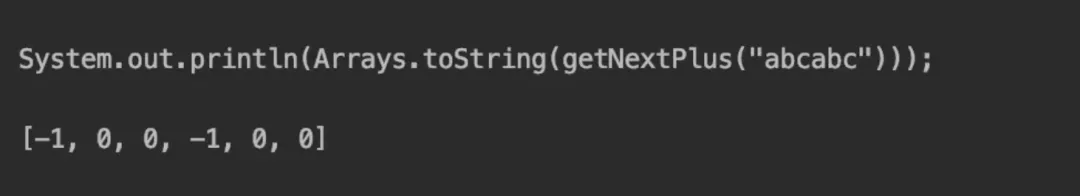/*****
* Java代码实现KMP模式匹配
*
* @param stringS 主串S
* @param stringT 模式串T
*/
public boolean match(String stringS, String stringT) {
char[] charsT = stringT.toCharArray();
int[] next = getNextPlus(stringT);
int j = 0;
for (int i = 0, sizeI = charsS.length; i < sizeI; i ++) {
// 此处0 > j其实就是针对next[j]=-1的情况，跳过主串当前字符的对比
if (0 > j || charsT[j] == charsS[i]) {
j ++;
} else {
j = next[j];
i --; // 实现继续对比主串的同一个位置的字符
}
if (charsT.length == j) {
return true;
}
}
return false;
}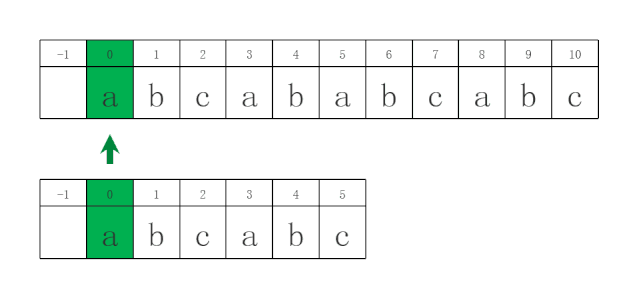/   结束语   /

PS: 如本文对您有疑惑，可加QQ：1752338621 进行讨论。

0
0